Updated by class11maths on Mar 04, 2022
REPORTclass11maths
Owner
14 items   3 followers   38 votes   3 views

# Class 11 Maths IIT JEE Mains

Class 11 Maths is a basics for students intended to go for Engineering studies like IIT JEE Mains, other entrances, boards, and higher studies in Mathematics. It covers all the basic to advance concepts, each NCERT solutions & important examples and much more.

1

## NCERT Exercise 1.1 & 1.2 Chapter 1 Sets Class 11 Maths IIT JEE Mains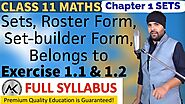High Quality Video Lecture explaining in detail about Sets, Roster Form, Set-builder form, and questions related to NCERT Exercise 1.1 & 1.2 of Sets Class 11 Maths IIT JEE Mains explained by expert teacher.

2

## Full Syllabus of Class 11 Maths | Mathyug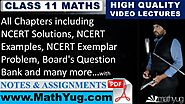Mathyug provides detailed video lectures and study material of Class 11 Maths having explanations from basic to advanced concepts, each NCERT exercise questions, important questions, supplementary exercise questions, important examples and Board's Question Bank that are arranged within assignments.

3

## Exercise 3.1 Trigonometric Functions Class 11 Maths IIT JEE Mains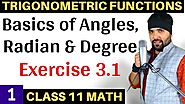In this video lecture, you will learn in detail Basics of Angles, Radian & Degree and NCERT Solutions for Exercise 3.1 Trigonometric Functions Class 11 Maths IIT JEE Mains explained by expert teacher.

4

## Exercise 5.2 Complex Numbers Class 11 Maths IIT JEE Mains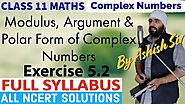High Quality Video Lecture explaining in detail about Modulus, Argument & Polar Form of Complex Numbers and questions related to NCERT Exercise 5.2 of Complex Numbers Class 11 Maths IIT JEE Mains explained by expert teacher.

5

## Exercise 2.1 Relations and Functions Class 11 Maths IIT JEE Mains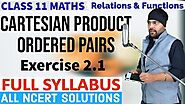High Quality Video Lecture explaining in detail about Cartesian Product, Ordered Pairs and questions related to NCERT Exercise 2.1 of Relations and Functions Class 11 Maths IIT JEE Mains explained by expert teacher.

6

## Exercise 4.1 Principle of Mathematical Induction Class 11 Maths IIT JEE Mains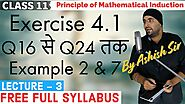High Quality Video Lecture explaining in detail about the examples and questions related to NCERT Exercise 4.1 of Principle of Mathematical Induction Class 11 Maths explained by expert teacher.

7

## NCERT Exercise 8.1 Binomial Theorem Class 11 Maths IIT JEE Mains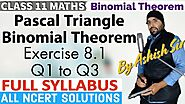High Quality Video Lecture explaining in detail about Pascal Triangle, Binomial Theorem and questions related to NCERT Solutions for Exercise 8.1 of Binomial Theorem Class 11 Maths IIT JEE Mains explained by expert teacher.

8

## Miscellaneous Exercise of Straight Lines Class 11 Maths IIT JEE Mains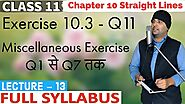High Quality Video Lecture explaining in detail about questions related to NCERT Solutions for Exercise 10.3 and Miscellaneous Exercise of NCERT Chapter 10 Straight Lines Class 11 Maths IIT JEE Mains explained by expert teacher.

9

## Basics of Limits and Derivatives Class 11 Maths IIT JEE Mains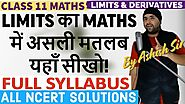High Quality Video Lecture explaining in detail about the basics and meaning of Limits and NCERT Solutions of Limits and Derivatives Class 11 Maths IIT JEE Mains explained by expert teacher.

10

## NCERT Chapter 16 Probability Class 11 Maths IIT JEE Mains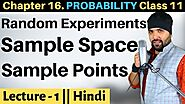High Quality Video Lecture explaining in detail about Sample Space and Sample Points with random experiments and questions related to NCERT Solutions for Chapter 16 Probability Class 11 Maths IIT JEE Mains explained by expert teacher.

11

## Miscellaneous Exercise -Sequences And Series Class 11 Maths IIT JEE Mains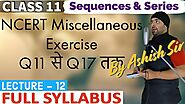High Quality Video Lecture explaining in detail about questions related to NCERT Solutions for Miscellaneous Exercise of NCERT Chapter 11 Sequences and Series Class 11 Maths IIT JEE Mains explained by expert teacher.

12

## NCERT Exercise 3.2 Trigonometric Functions Class 11 Maths IIT JEE Mains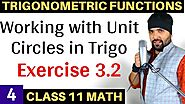Detailed explanation of Working with Unit Circles in Trigonometry and questions related to NCERT Solutions for Exercise 3.2 Trigonometric Functions Class 11 Maths IIT JEE Mains explained by expert teacher.

13

## Exercise 3.3 Trigonometric Functions Class 11 Maths IIT JEE Mains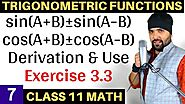Detailed explanation of Derivation and questions related to NCERT Solutions for Exercise 3.3 Trigonometric Functions Class 11 Maths IIT JEE Mains explained by expert teacher.

14

## Student Enrollment for Class 10 Maths, Class 11 Maths & Class 12 Maths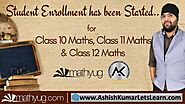Student Enrollment for Class 10 Maths, Class 11 Maths & Class 12 Maths has been started on both platforms 'Ashish Kumar - Let's Learn' and 'MathYug'.